Introduction to R and data exploration

Martijn Wieling
University of Groningen

This lecture

• RStudio and R
• R as calculator
• Variables
• Functions and help
• Importing data in R in a dataframe
• Accessing rows and columns
• Adding columns to the data
• Goal of statistics
• Data exploration (descriptive statistics)
• Numerical measures
• Visual exploration

Our tool: RStudio (frontend to R)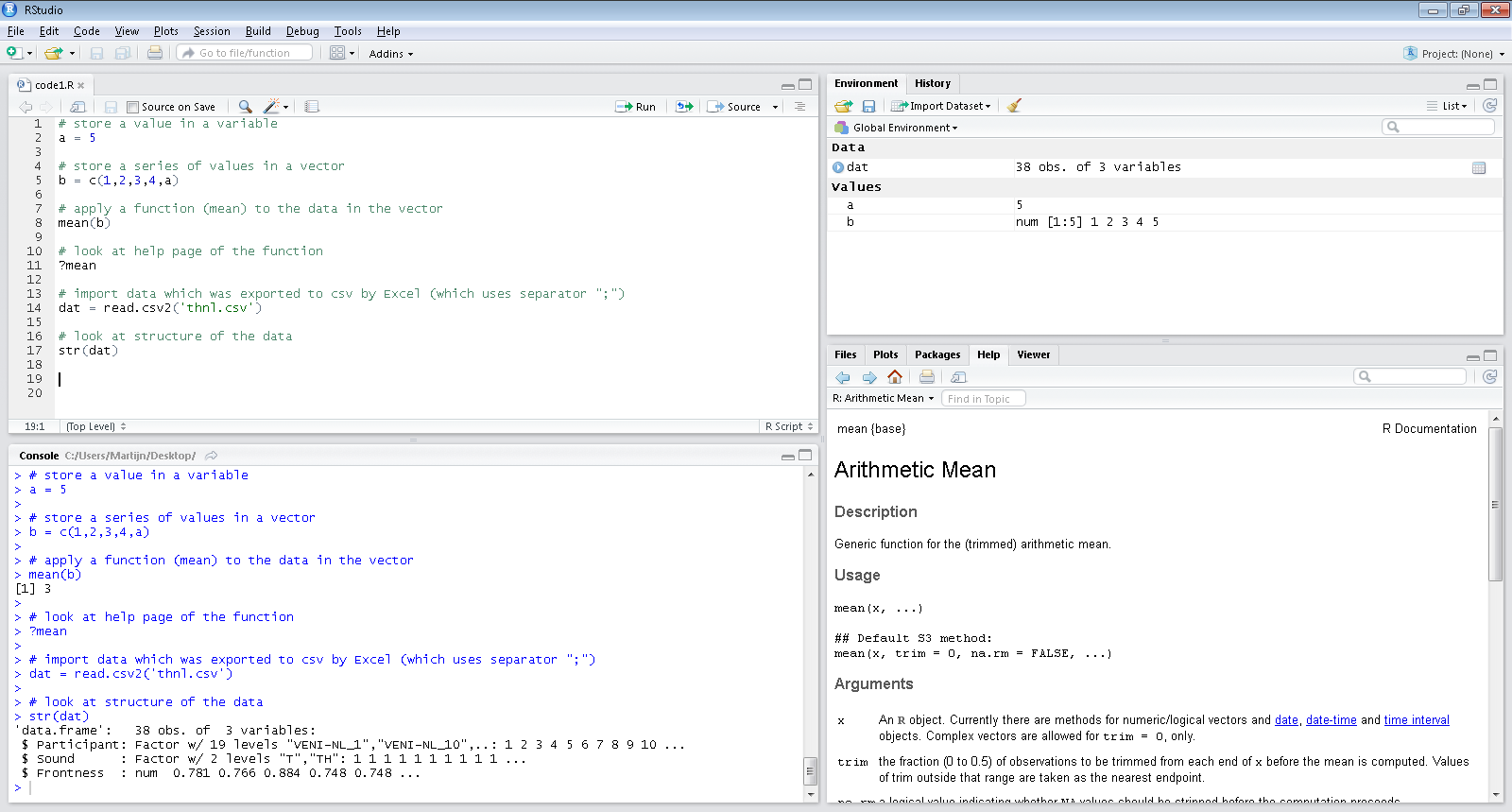RStudio: quick overview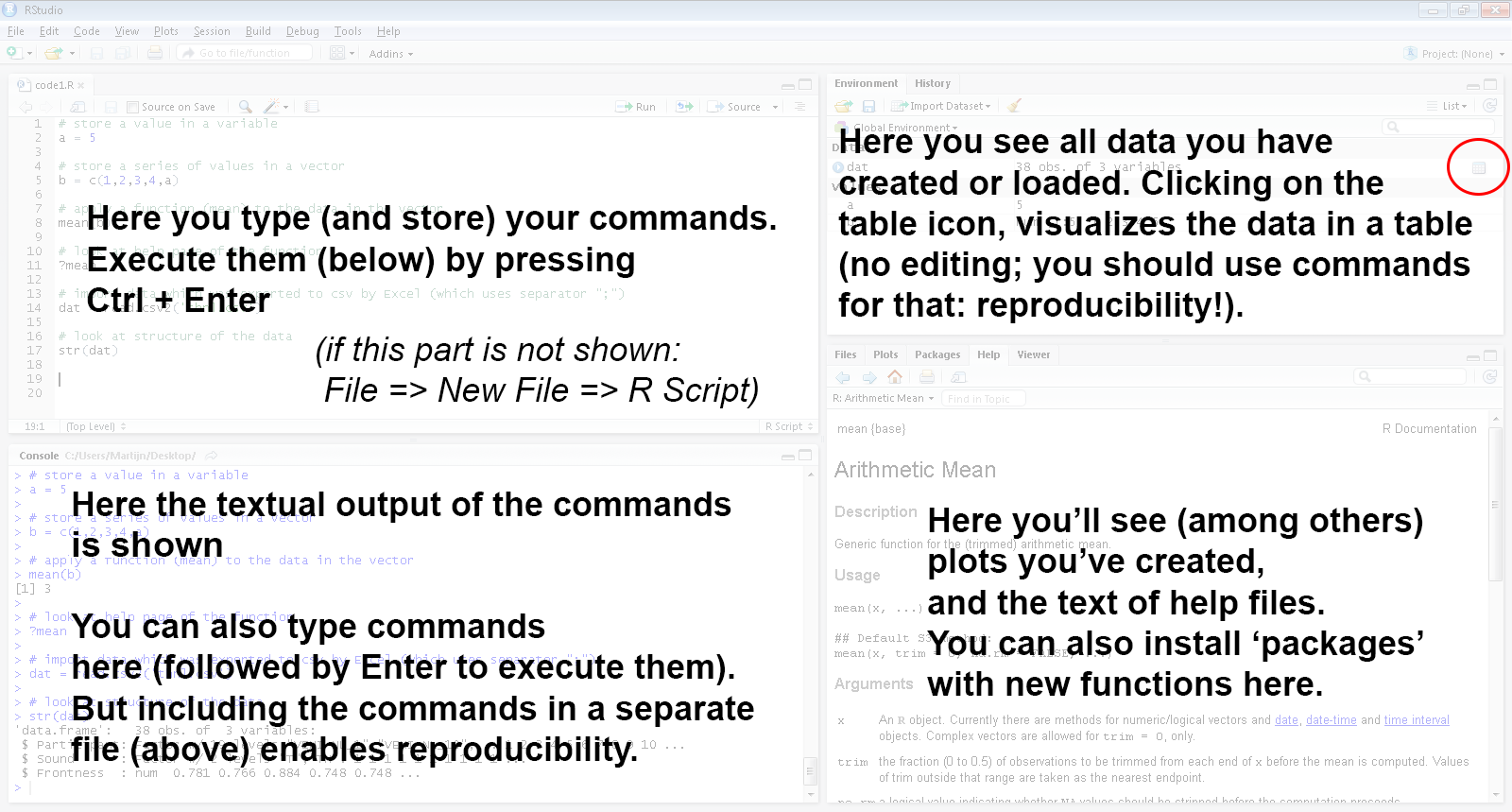Basic functionality: R as calculator

# Addition (this is a comment: preceded by '#')
5 + 5
#  10
# Multiplication
5 * 3
#  15
# Division
5/3
#  1.6667

Basic functionality: using variables

a <- 5  # store a single value; instead of '<-' you can also use '='
a  # display the value
#  5
b <- c(2, 4, 6, 7, 8)  # store a series of values in a vector
b
#  2 4 6 7 8
b <- a  # assign value 5 (stored in 'a') to the 4th element of vector b
b <- NA  # assign NA (missing) to the first element of vector b
b <- b * 10  # multiply all values in vector b with 10
b
#  NA 40 60 50 80

Basic functionality: using functions

mn <- mean(b)  # calculating the mean and storing in variable mn
mn
#  NA
# mn is NA (missing) as one of the values is missing
mean(b, na.rm = TRUE)  # we can use the function parameter na.rm to ignore NAs
#  57.5
# But which parameters does a function have: use help!
help(mean)  # alternatively: ?mean

Basic functionality: a help fileTry it yourself!

• There are many resources for R which you can easily find online
• Here we use "swirl" an online platform for interactive R courses
• Start RStudio, install and start swirl:
install.packages("swirl", repos = "http://cran.rstudio.com/")
library(swirl)
swirl()
• Follow the prompts and install the course R programming: The basics of programming in R
• Choose that course to start with and finish Lesson 1 of that course

Getting data into R: exporting a data set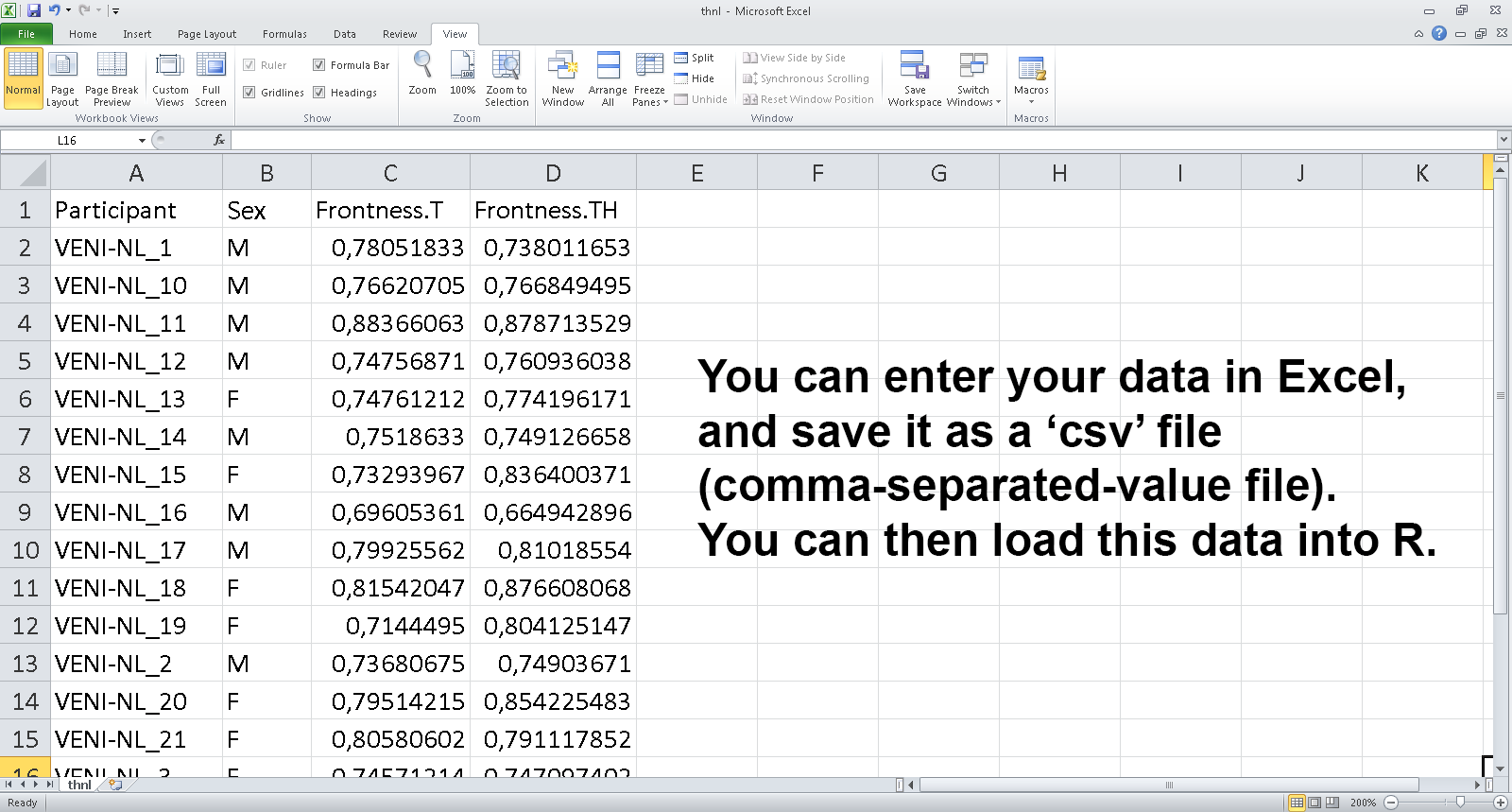Getting data into R: importing a data set

setwd("C:/Users/Martijn/Desktop/Statistics/Intro-R")  # set working directory
str(dat)  # shows structure of the data frame dat (note: wide format)
# 'data.frame': 19 obs. of  4 variables:
#  \$ Participant : chr  "VENI-NL_1" "VENI-NL_10" "VENI-NL_11" "VENI-NL_12" ...
#  \$ Gender      : chr  "M" "M" "M" "M" ...
#  \$ Frontness.T : num  0.781 0.766 0.884 0.748 0.748 ...
#  \$ Frontness.TH: num  0.738 0.767 0.879 0.761 0.774 ...
dim(dat)  # number of rows and columns of data set
#  19  4

Investigating imported data set: using head

head(dat)  # show first few rows of dat
#   Participant Gender Frontness.T Frontness.TH
# 1   VENI-NL_1      M     0.78052      0.73801
# 2  VENI-NL_10      M     0.76621      0.76685
# 3  VENI-NL_11      M     0.88366      0.87871
# 4  VENI-NL_12      M     0.74757      0.76094
# 5  VENI-NL_13      M     0.74761      0.77420
# 6  VENI-NL_14      M     0.75186      0.74913

Investigating imported data set: using RStudio viewer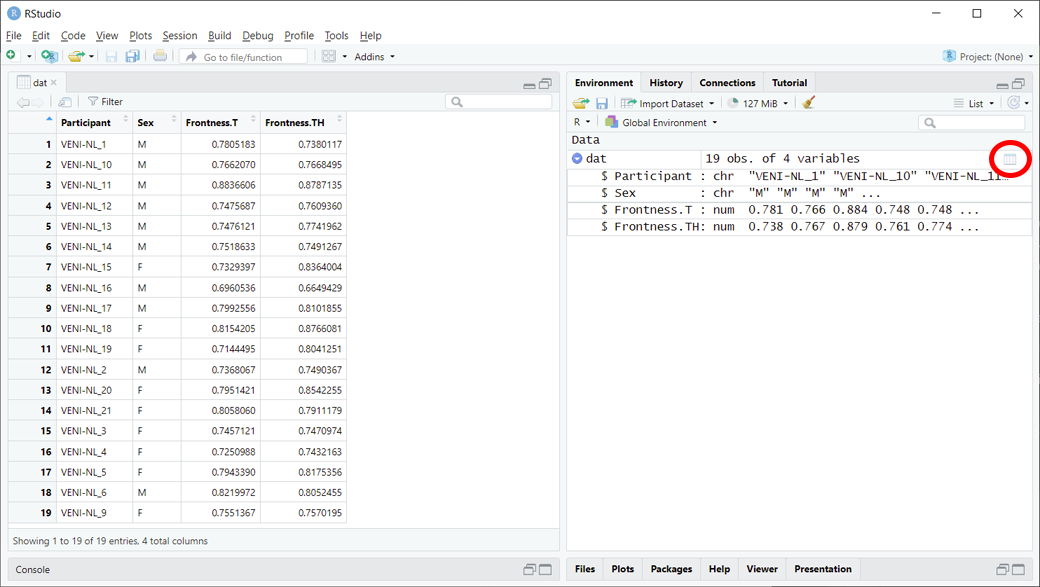Subsetting the data: indices and names

dat[1, ]  # values in first row
#   Participant Gender Frontness.T Frontness.TH
# 1   VENI-NL_1      M     0.78052      0.73801
dat[1:2, c(2, 3)]  # values of first two rows for second and third column
#   Gender Frontness.T
# 1      M     0.78052
# 2      M     0.76621
dat[c(1, 2, 3), "Participant"]  # values of first three rows for column 'Participant'
#  "VENI-NL_1"  "VENI-NL_10" "VENI-NL_11"
tmp <- dat[5:8, c(1, 3)]  # store columns 1 and 3 for rows 5 to 8 in tmp

Subsetting the data: conditional indexing

tmp <- dat[dat\$Gender == "M", ]  # only observations for male participants
head(tmp, n = 2)  # show first two rows
#   Participant Gender Frontness.T Frontness.TH
# 1   VENI-NL_1      M     0.78052      0.73801
# 2  VENI-NL_10      M     0.76621      0.76685
# more advanced subsetting: include rows for which frontness for the T sound is higher
# than 0.74 AND participant is either 1 or 2 N.B. use '|' instead of '&' for logical
# OR
dat[dat\$Frontness.T > 0.74 & dat\$Participant %in% c("VENI-NL_1", "VENI-NL_2"), ]
#   Participant Gender Frontness.T Frontness.TH
# 1   VENI-NL_1      M     0.78052      0.73801

Question 5

# new column Diff containing difference between TH and T positions
dat\$Diff <- dat\$Frontness.TH - dat\$Frontness.T

# new column DiffClass, initially all observations set to TH0
dat\$DiffClass <- "TH0"

# observations with Diff larger than 0.02 are categorized as TH1, negative as TH-
dat[dat\$Diff > 0.02, ]\$DiffClass <- "TH1"
dat[dat\$Diff < 0, ]\$DiffClass <- "TH-"

dat\$DiffClass <- factor(dat\$DiffClass)  # convert string variable to factor

#   Participant Gender Frontness.T Frontness.TH        Diff DiffClass
# 1   VENI-NL_1      M     0.78052      0.73801 -0.04250668       TH-
# 2  VENI-NL_10      M     0.76621      0.76685  0.00064245       TH0

Try it yourself!

• Run swirl() and finish the following lessons of the R Programming course:
• Lesson 6: Subsetting vectors
• Lesson 12: Looking at data

Statistics

• Goal of statistics is to gain understanding from data
• Descriptive statistics (this lecture): describe data without further conclusions
• Inferential statistics: describe data (sample) and its relation to larger group (population)

Numerical variables: central tendency and spread

mean(dat\$Diff)  # mean
#  0.016263
median(dat\$Diff)  # median
#  0.01093
min(dat\$Diff)  # minimum value
#  -0.042507
max(dat\$Diff)  # maximum value
#  0.10346

sd(dat\$Diff)  # or: sqrt((1/(length(dat\$Diff)-1)) * sum((dat\$Diff - mean(dat\$Diff))^2))
#  0.038213
var(dat\$Diff)  # or: sd(dat\$Diff)^2
#  0.0014603
quantile(dat\$Diff)  # quantiles
#         0%        25%        50%        75%       100%
# -0.0425067 -0.0038419  0.0109299  0.0248903  0.1034607
summary(dat\$Diff)  # summary
#     Min.  1st Qu.   Median     Mean  3rd Qu.     Max.
# -0.04251 -0.00384  0.01093  0.01626  0.02489  0.10346

Categorical variables: frequency tables

table(dat\$Gender)
#
#  F  M
#  9 10
with(dat, table(Gender))  # alternative
# Gender
#  F  M
#  9 10
table(dat\$DiffClass)
#
# TH- TH0 TH1
#   6   7   6

Exploring relationships between pairs of variables

# correlation: relation between two numerical variables
cor(dat\$Frontness.T, dat\$Frontness.TH)
#  0.71054
# crosstable: relation between two categorical variables
table(dat\$Gender, dat\$DiffClass)  # or: with(dat, table(Gender,DiffClass))
#
#     TH- TH0 TH1
#   F   1   3   5
#   M   5   4   1
# means per category: relation between numerical and categorical variable
c(mean(dat[dat\$Gender == "M", ]\$Diff), mean(dat[dat\$Gender == "F", ]\$Diff))
#  -0.0034299  0.0381446

Data exploration with visualization

• Many basic visualization options are available in R
• boxplot() for a boxplot
• hist() for a histogram
• qqnorm() and qqline() for a quantile-quantile plot
• plot() for many types of plots (scatter, line, etc.)
• barplot() for a barplot (plotting frequencies)

Exploring numerical variables: box plot

par(mfrow = c(1, 2))  # set graphics option: 2 graphs side-by-side
boxplot(dat\$Diff, main = "Difference")  # boxplot of difference values
boxplot(dat[, c("Frontness.T", "Frontness.TH")])  # frontness per group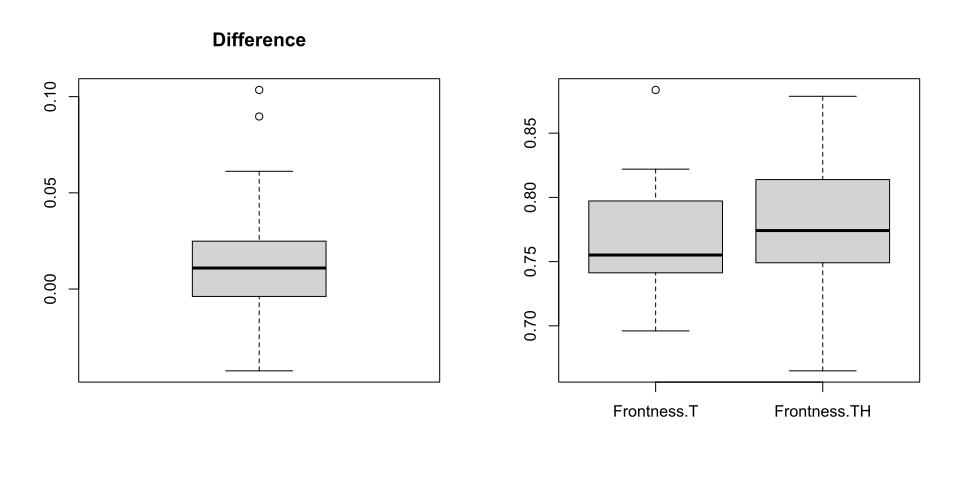Exploring numerical variables: histogram

hist(dat\$Diff, main = "Difference histogram")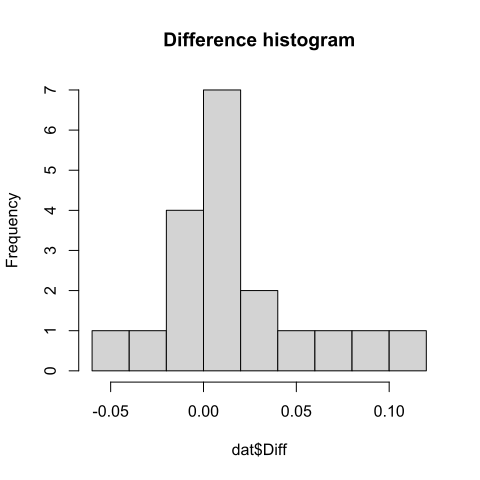Exploring numerical variables: Q-Q plot

qqnorm(dat\$Diff)  # plot actual values vs. theoretical quantiles
qqline(dat\$Diff)  # plot reference line of normal distribution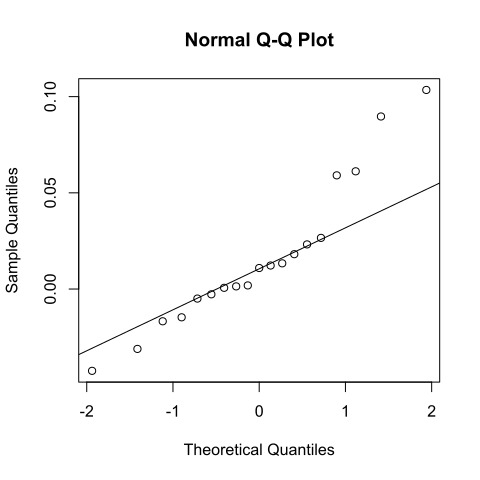Exploring numerical relations: scatter plot

plot(dat\$Frontness.T, dat\$Frontness.TH, col = "blue")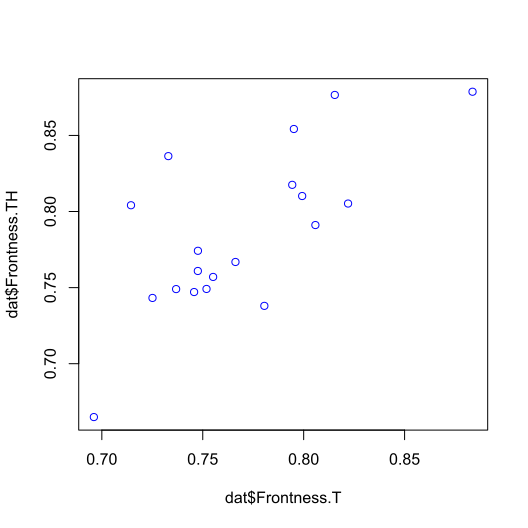Visualizing categorical variables (frequencies): bar plot

counts <- table(dat\$Gender)  # frequency table for gender
barplot(counts, ylim = c(0, 15))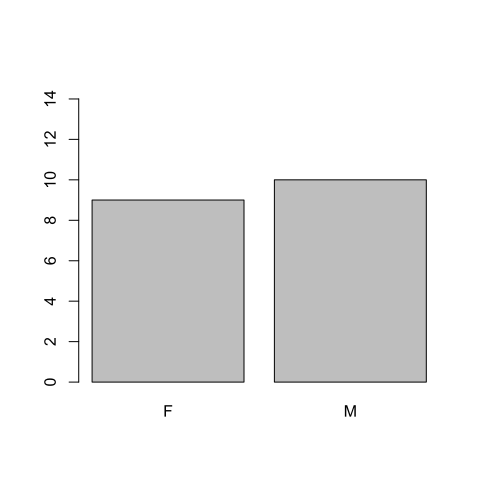Exploring categorical relations: segmented bar plot

counts <- table(dat\$Gender, dat\$DiffClass)
barplot(counts, col = c("pink", "lightblue"), legend = rownames(counts), ylim = c(0, 10))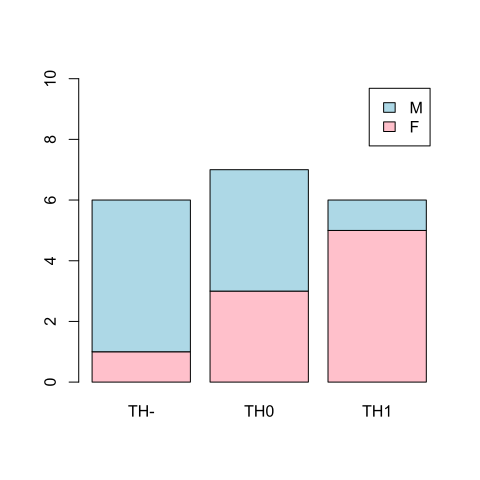Try it yourself!

• Run swirl() and finish the following lesson of the R Programming course:
• Lesson 15: Base graphics

Recap

• In this lecture, we've covered the basics of R
• Now you should be able (with help of this presentation) to use R for:
• Data manipulation, exploration and visualization
• Associated lab session and additional swirl resources: